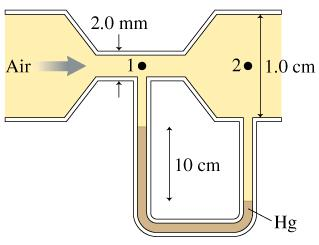# Problem: Air flows through the tube shown in the figure. Assume that air is an ideal fluid. ﻿What is the air speed v1 at point 1?

###### FREE Expert Solution

Bernoulli's equation:

$\overline{){{\mathbf{P}}}_{{\mathbf{1}}}{\mathbf{+}}\frac{\mathbf{1}}{\mathbf{2}}{\mathbf{\rho }}{{{\mathbf{v}}}_{{\mathbf{1}}}}^{{\mathbf{2}}}{\mathbf{+}}{{\mathbf{h}}}_{{\mathbf{1}}}{\mathbf{\rho }}{\mathbf{g}}{\mathbf{=}}{{\mathbf{P}}}_{{\mathbf{2}}}{\mathbf{+}}\frac{\mathbf{1}}{\mathbf{2}}{\mathbf{\rho }}{{\mathbf{v}}_{\mathbf{2}}}^{{\mathbf{2}}}{\mathbf{+}}{{\mathbf{h}}}_{{\mathbf{2}}}{\mathbf{\rho }}{\mathbf{g}}}$ where ρ is the density of the moving fluid, h is the difference in height between the two points.

Continuity equation:

$\overline{){\mathbf{Q}}{\mathbf{=}}{{\mathbf{A}}}_{{\mathbf{1}}}{{\mathbf{v}}}_{{\mathbf{1}}}{\mathbf{=}}{{\mathbf{A}}}_{{\mathbf{2}}}{{\mathbf{v}}}_{{\mathbf{2}}}{\mathbf{=}}{{\mathbf{A}}}_{{\mathbf{n}}}{{\mathbf{v}}}_{{\mathbf{n}}}}$

Pressure in liquids:

$\overline{){\mathbit{P}}{\mathbf{=}}{\mathbit{\rho }}{\mathbit{g}}{\mathbit{h}}}$

We'll use Bernoulli's equation to solve for the velocity.

The pressure is greater in the wider cross section. P2 is greater than P1.

The elevation at points 1 and 2 is equal, h1 = h2. Thus, we can drop the terms h1ρairg and h2ρairg.

Using Bernoulli's equation:

85% (325 ratings)###### Problem Details

Air flows through the tube shown in the figure. Assume that air is an ideal fluid. ﻿What is the air speed v1 at point 1?